In my last post I discussed using `coefplot` on `glmnet` models and in particular discussed a brand new function, `coefpath`, that uses `dygraphs` to make an interactive visualization of the coefficient path.

Another new capability for version 1.2.5 of `coefplot` is the ability to show coefficient plots from `xgboost` models. Beyond fitting boosted trees and boosted forests, `xgboost` can also fit a boosted Elastic Net. This makes it a nice alternative to `glmnet` even though it might not have some of the same user niceties.

To illustrate, we use the same data as our previous post.

First, we load the packages we need and note the version numbers.

``````# list the packages that we load
# alphabetically for reproducibility
packages <- c('caret', 'coefplot', 'DT', 'xgboost')
# call library on each package
purrr::walk(packages, library, character.only=TRUE)

# they are listed here for complete documentation
packagesColon <- c('dplyr', 'dygraphs', 'knitr', 'magrittr', 'purrr', 'tibble', 'useful')``````
``````versions <- c(packages, packagesColon) %>%
purrr::map(packageVersion) %>%
purrr::map_chr(as.character)
packageDF <- tibble::data_frame(Package=c(packages, packagesColon), Version=versions) %>%
dplyr::arrange(Package)
knitr::kable(packageDF)``````
Package Version
caret 6.0.78
coefplot 1.2.6
dplyr 0.7.4
DT 0.2
dygraphs 1.1.1.4
knitr 1.18
magrittr 1.5
purrr 0.2.4
tibble 1.4.2
useful 1.2.3
xgboost 0.6.4

Then, we read the data. The data are available at http://www.jaredlander.com/data/manhattan_Train.rds with the CSV version at data.world. We also get validation data which is helpful when fitting `xgboost` mdoels.

``````manTrain <- readRDS(url('http://www.jaredlander.com/data/manhattan_Train.rds'))

The data are about New York City land value and have many columns. A sample of the data follows. There’s an odd bug where you have to click on one of the column names for the data to display the actual data.

``````datatable(manTrain %>% dplyr::sample_n(size=1000), elementId='TrainingSampled',
rownames=FALSE,
options=list(
scroller=TRUE
))``````

While `glmnet` automatically standardizes the input data, `xgboost` does not, so we calculate that manually. We use `preprocess` from `caret` to compute the mean and standard deviation of each numeric column then use these later.

``preProc <- preProcess(manTrain, method=c('center', 'scale'))``

Just like with `glmnet`, we need to convert our `tbl` into an X (predictor) `matrix` and a Y (response) `vector`. Since we don’t have to worry about multicolinearity with `xgboost` we do not want to drop the baselines of `factor`s. We also take advantage of sparse matrices since that reduces memory usage and compute, even though this dataset is not that large.

In order to build the `matrix` and `vector` we need a `formula`. This could be built programmatically, but we can just build it ourselves. The response is `TotalValue`.

``````valueFormula <- TotalValue ~ FireService + ZoneDist1 + ZoneDist2 +
Class + LandUse + OwnerType + LotArea + BldgArea + ComArea + ResArea +
OfficeArea + RetailArea + NumBldgs + NumFloors + UnitsRes + UnitsTotal +
LotDepth + LotFront + BldgFront + LotType + HistoricDistrict + Built +
Landmark``````
``````manX <- useful::build.x(valueFormula, data=predict(preProc, manTrain),
# do not drop the baselines of factors
contrasts=FALSE,
# use a sparse matrix
sparse=TRUE)

manY <- useful::build.y(valueFormula, data=manTrain)

manX_val <- useful::build.x(valueFormula, data=predict(preProc, manVal),
# do not drop the baselines of factors
contrasts=FALSE,
# use a sparse matrix
sparse=TRUE)

manY_val <- useful::build.y(valueFormula, data=manVal)``````

There are two functions we can use to fit `xgboost` models, the eponymous `xgboost` and `xgb.train`. When using `xgb.train` we first store our X and Y matrices in a special `xgb.DMatrix` object. This is not a necessary step, but makes things a bit cleaner.

``````manXG <- xgb.DMatrix(data=manX, label=manY)
manXG_val <- xgb.DMatrix(data=manX_val, label=manY_val)``````

We are now ready to fit a model. All we need to do to fit a linear model instead of a tree is set `booster='gblinear'` and `objective='reg:linear'`.

``````mod1 <- xgb.train(
# the X and Y training data
data=manXG,
# use a linear model
booster='gblinear',
# minimize the a regression criterion
objective='reg:linear',
# use MAE as a measure of quality
eval_metric=c('mae'),
# boost for up to 500 rounds
nrounds=500,
# print out the eval_metric for both the train and validation data
watchlist=list(train=manXG, validate=manXG_val),
# print eval_metric every 10 rounds
print_every_n=10,
# if the validate eval_metric hasn't improved by this many rounds, stop early
early_stopping_rounds=25,
# penalty terms for the L2 portion of the Elastic Net
lambda=10, lambda_bias=10,
# penalty term for the L1 portion of the Elastic Net
alpha=900000000,
# randomly sample rows
subsample=0.8,
# randomly sample columns
col_subsample=0.7,
# set the learning rate for gradient descent
eta=0.1
)``````
``````##   train-mae:1190145.875000    validate-mae:1433464.750000
## Multiple eval metrics are present. Will use validate_mae for early stopping.
## Will train until validate_mae hasn't improved in 25 rounds.
##
##  train-mae:938069.937500 validate-mae:1257632.000000
##  train-mae:932016.625000 validate-mae:1113554.625000
##  train-mae:931483.500000 validate-mae:1062618.250000
##  train-mae:931146.750000 validate-mae:1054833.625000
##  train-mae:930707.312500 validate-mae:1062881.375000
##  train-mae:930137.375000 validate-mae:1077038.875000
## Stopping. Best iteration:
##  train-mae:931146.750000 validate-mae:1054833.625000``````

The best fit was arrived at after 41 rounds. We can see how the model did on the train and validate sets using `dygraphs`.

``dygraphs::dygraph(mod1\$evaluation_log)``

We can now plot the coefficients using `coefplot`. Since `xgboost` does not save column names, we specify it with `feature_names=colnames(manX)`. Unlike with `glmnet` models, there is only one penalty so we do not need to specify a specific penalty to plot.

``coefplot(mod1, feature_names=colnames(manX), sort='magnitude')``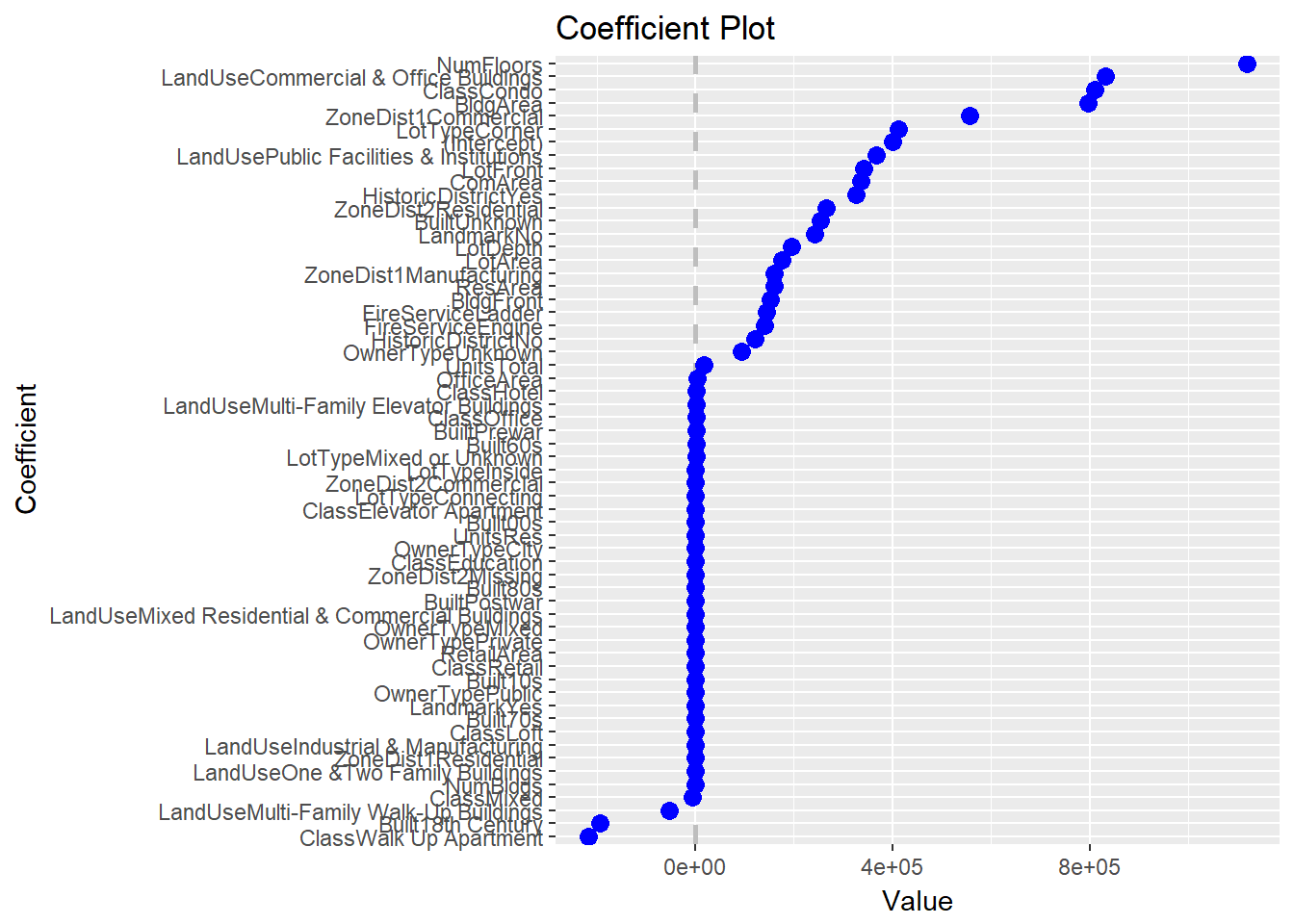This is another nice addition to `coefplot` utilizing the power of `xgboost`.

#### Related PostsJared Lander is the Chief Data Scientist of Lander Analytics a New York data science firm, Adjunct Professor at Columbia University, Organizer of the New York Open Statistical Programming meetup and the New York and Washington DC R Conferences and author of R for Everyone.I’m a big fan of the Elastic Net for variable selection and shrinkage and have given numerous talks about it and its implementation, `glmnet`. In fact, I will even have a DataCamp course about glmnet coming out soon.

As a side note, I used to pronounce it g-l-m-net but after having lunch with one of its creators, Trevor Hastie, I learn it is pronounced glimnet.

`coefplot` has long supported `glmnet` via a standard coefficient plot but I recently added some functionality, so let’s take a look. As we go through this, please pardon the `htmlwidgets` in iframes.

First, we load packages. I am now fond of using the following syntax for loading the packages we will be using.

``````# list the packages that we load
# alphabetically for reproducibility
packages <- c('coefplot', 'DT', 'glmnet')
# call library on each package
purrr::walk(packages, library, character.only=TRUE)

# they are listed here for complete documentation
packagesColon <- c('dplyr', 'knitr', 'magrittr', 'purrr', 'tibble', 'useful')``````

The versions can then be displayed in a table.

``````versions <- c(packages, packagesColon) %>%
purrr::map(packageVersion) %>%
purrr::map_chr(as.character)
packageDF <- tibble::data_frame(Package=c(packages, packagesColon), Version=versions) %>%
dplyr::arrange(Package)
knitr::kable(packageDF)``````
Package Version
coefplot 1.2.5.1
dplyr 0.7.4
DT 0.2
glmnet 2.0.13
knitr 1.18
magrittr 1.5
purrr 0.2.4
tibble 1.4.1
useful 1.2.3

First, we read some data. The data are available at http://www.jaredlander.com/data/manhattan_Train.rds with the CSV version at data.world.

``manTrain <- readRDS(url('http://www.jaredlander.com/data/manhattan_Train.rds'))``

The data are about New York City land value and have many columns. A sample of the data follows.

``````datatable(manTrain %>% dplyr::sample_n(size=100), elementId='DataSampled',
rownames=FALSE,
options=list(
scroller=TRUE,
scrollY=300
))``````

In order to use `glmnet` we need to convert our `tbl` into an X (predictor) `matrix` and a Y (response) `vector`. Since we don’t have to worry about multicolinearity with `glmnet` we do not want to drop the baselines of `factor`s. We also take advantage of sparse matrices since that reduces memory usage and compute, even though this dataset is not that large.

In order to build the `matrix` ad `vector` we need a `formula`. This could be built programmatically, but we can just build it ourselves. The response is `TotalValue`.

``````valueFormula <- TotalValue ~ FireService + ZoneDist1 + ZoneDist2 +
Class + LandUse + OwnerType + LotArea + BldgArea + ComArea + ResArea +
OfficeArea + RetailArea + NumBldgs + NumFloors + UnitsRes + UnitsTotal +
LotDepth + LotFront + BldgFront + LotType + HistoricDistrict + Built +
Landmark - 1``````

Notice the `- 1` means do not include an intercept since `glmnet` will do that for us.

``````manX <- useful::build.x(valueFormula, data=manTrain,
# do not drop the baselines of factors
contrasts=FALSE,
# use a sparse matrix
sparse=TRUE)

manY <- useful::build.y(valueFormula, data=manTrain)``````

We are now ready to fit a model.

``mod1 <- glmnet(x=manX, y=manY, family='gaussian')``

We can view a coefficient plot for a given value of `lambda` like this.

``coefplot(mod1, lambda=330500, sort='magnitude')``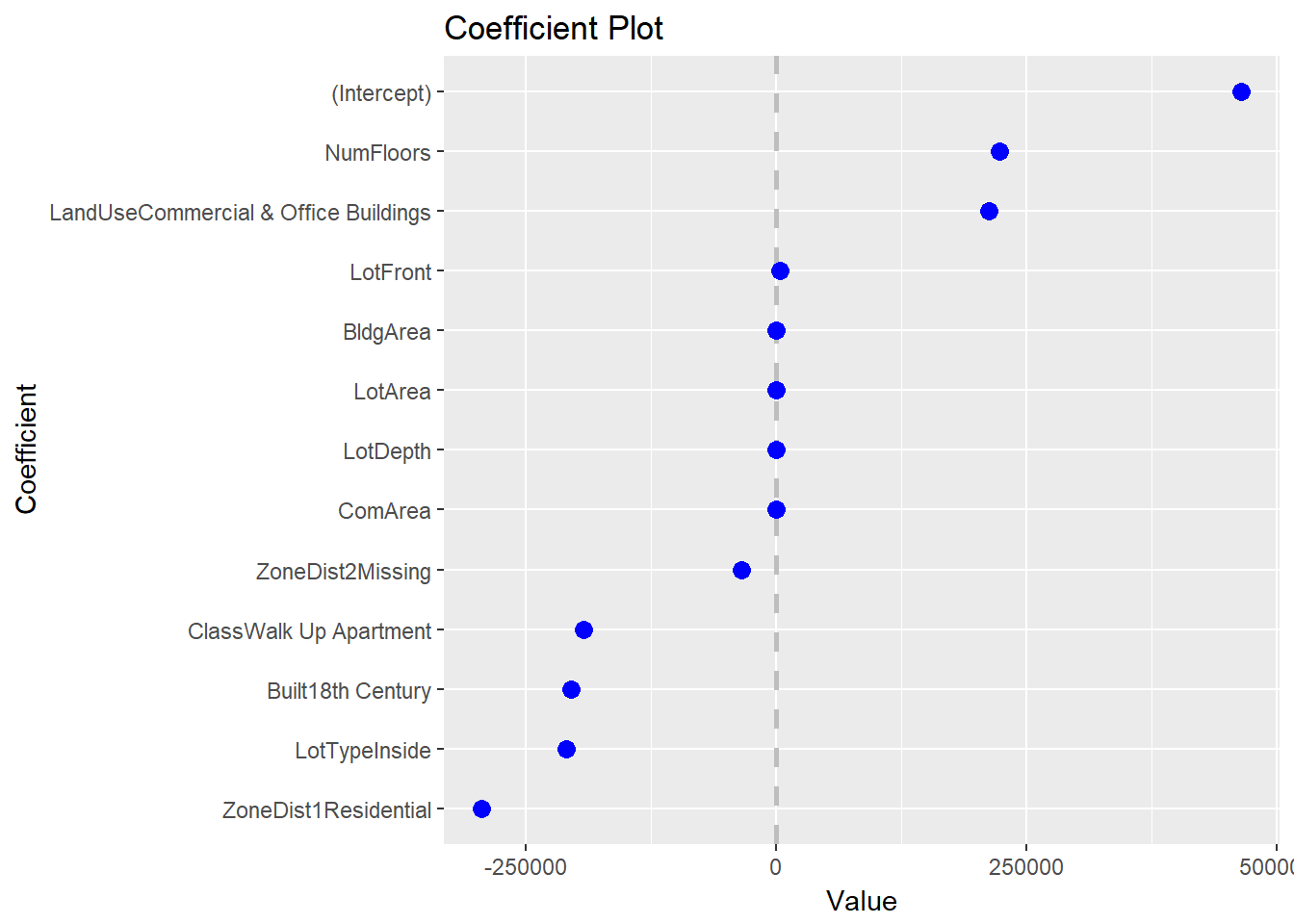A common plot that is built into the `glmnet` package it the coefficient path.

``plot(mod1, xvar='lambda', label=TRUE)``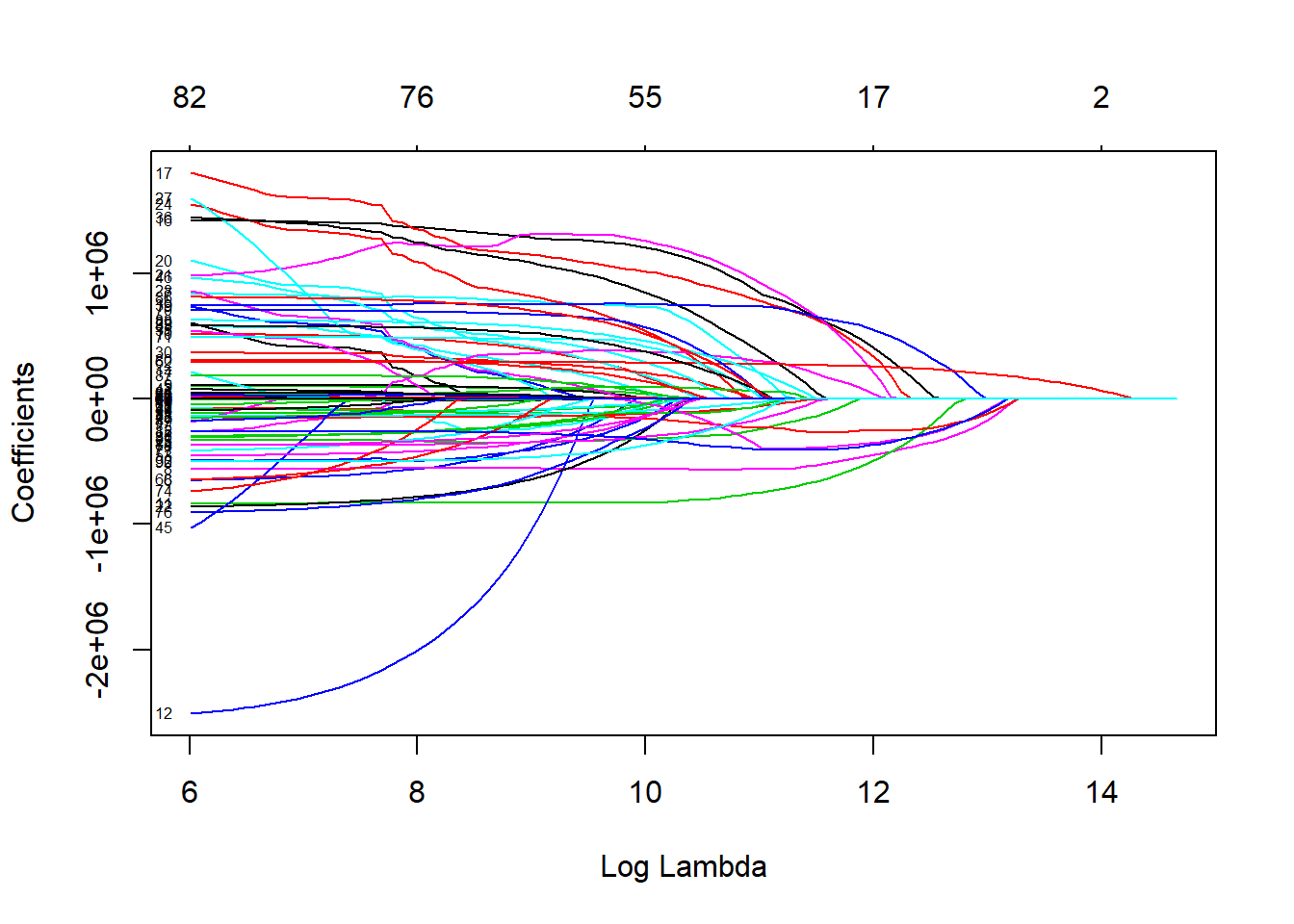This plot shows the path the coefficients take as `lambda` increases. They greater `lambda` is, the more the coefficients get shrunk toward zero. The problem is, it is hard to disambiguate the lines and the labels are not informative.

Fortunately, `coefplot` has a new function in Version 1.2.5 called `coefpath` for making this into an interactive plot using dygraphs.

``coefpath(mod1)``

While still busy this function provides so much more functionality. We can hover over lines, zoom in then pan around.

These functions also work with any value for `alpha` and for cross-validated models fit with `cv.glmnet`.

``mod2 <- cv.glmnet(x=manX, y=manY, family='gaussian', alpha=0.7, nfolds=5)``

We plot coefficient plots for both optimal `lambda`s.

``````# coefplot for the 1se error lambda
coefplot(mod2, lambda='lambda.1se', sort='magnitude')``````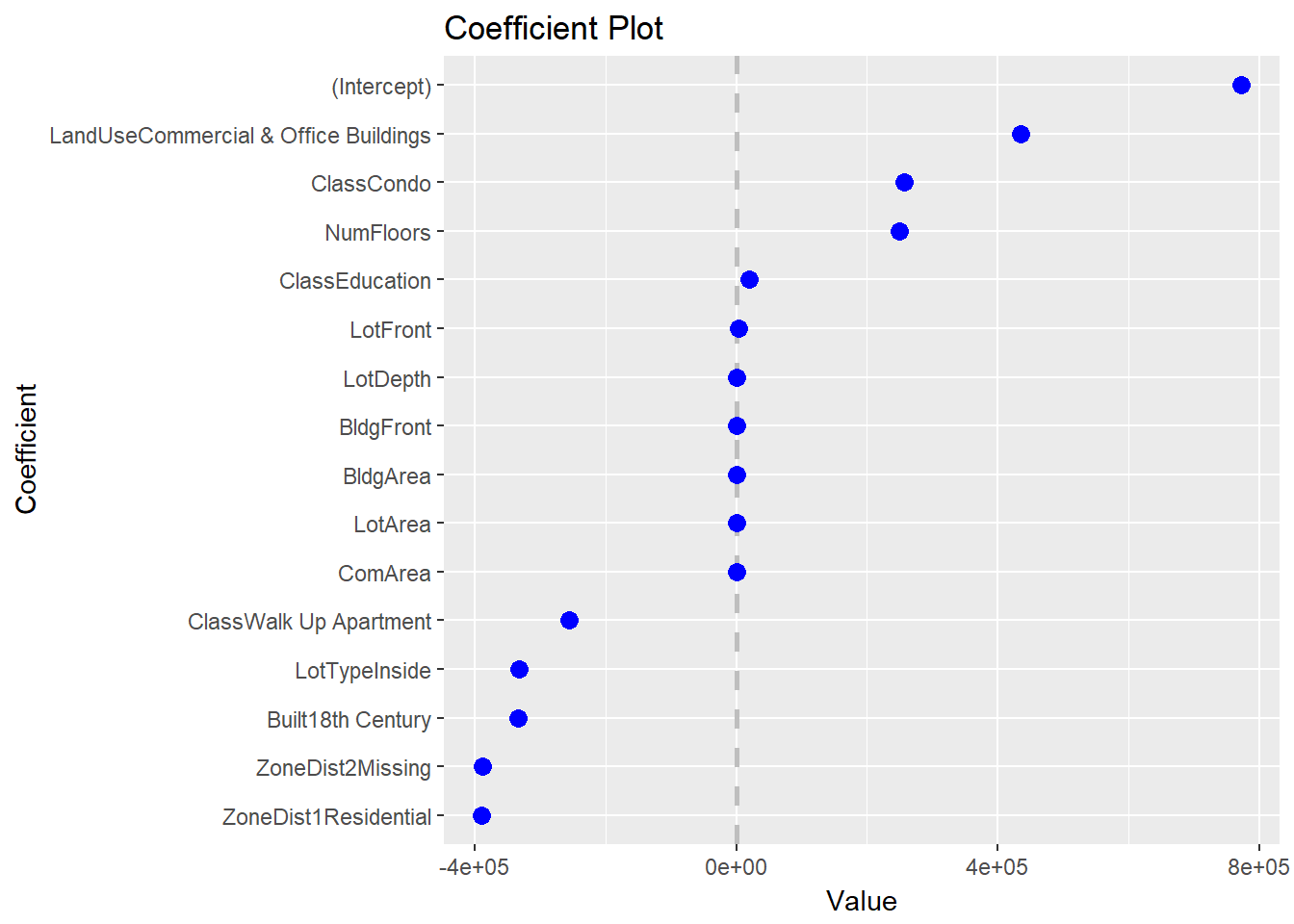``````# coefplot for the min error lambda
coefplot(mod2, lambda='lambda.min', sort='magnitude')``````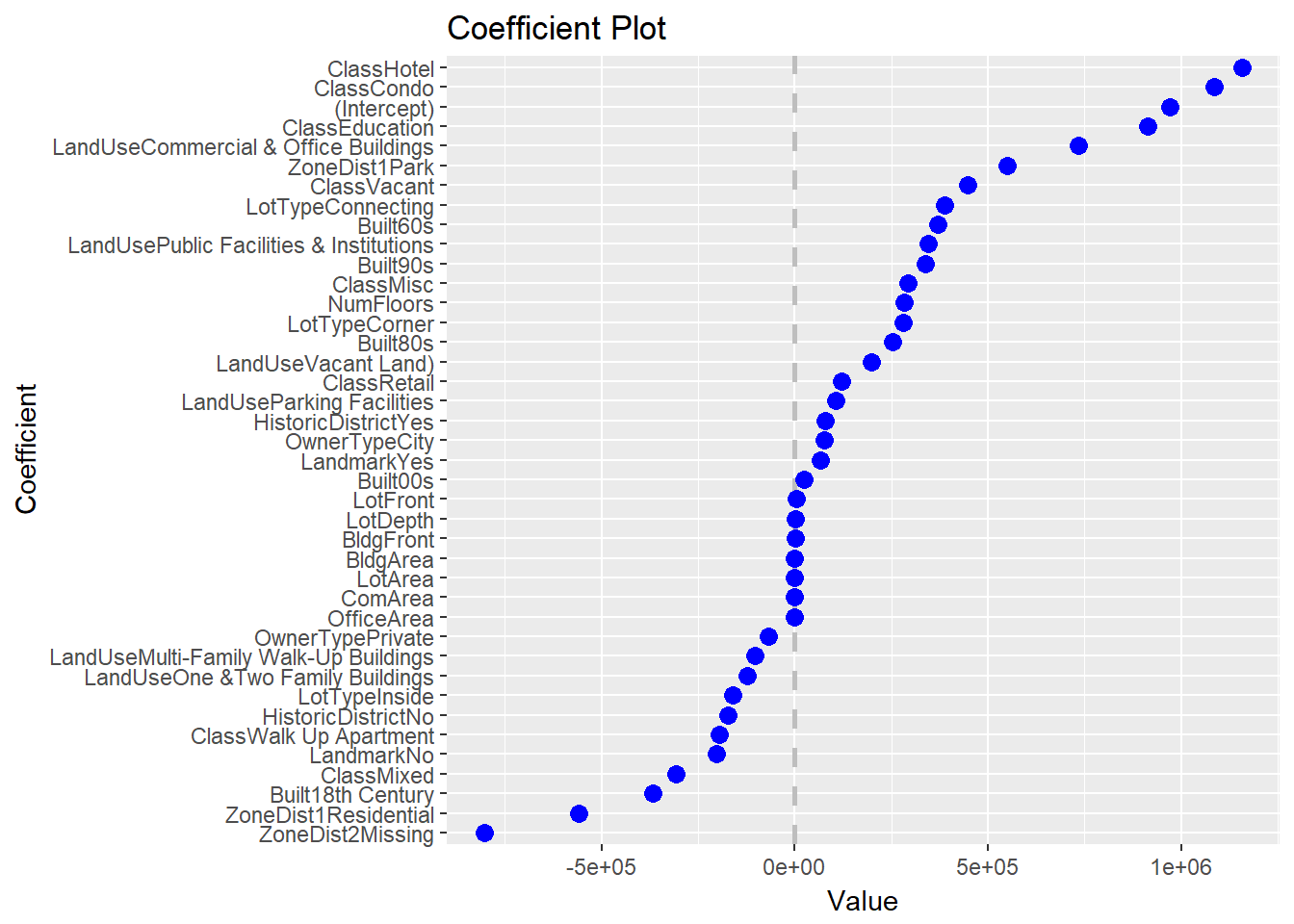The coefficient path is the same as before though the optimal `lambda`s are noted as dashed vertical lines.

``coefpath(mod2)``

While `coefplot` has long been able to plot coefficients from `glmnet` models, the new `coefpath` function goes a long way in helping visualize the paths the coefficients take as `lambda` changes.Jared Lander is the Chief Data Scientist of Lander Analytics a New York data science firm, Adjunct Professor at Columbia University, Organizer of the New York Open Statistical Programming meetup and the New York and Washington DC R Conferences and author of R for Everyone.An often requested feature for Hadley Wickham's ggplot2 package is the ability to vertically dodge points, lines and bars. There has long been a function to shift geoms to the side when the x-axis is categorical: position_dodge. However, no such function exists for vertical shifts when the y-axis is categorical. Hadley usually responds by saying it should be easy to build, so here is a hacky patch.

All I did was copy the old functions (geom_dodge, collide, pos_dodge and PositionDodge) and make them vertical by swapping y's with x's, height with width and vice versa. It's hacky and not tested but seems to work as I'll show below.

First the new functions:

``````require(proto)
``````
``````## Loading required package: proto
``````
``````collidev <- function(data, height = NULL, name, strategy, check.height = TRUE) {
if (!is.null(height)) {
if (!(all(c("ymin", "ymax") %in% names(data)))) {
data <- within(data, {
ymin <- y - height/2
ymax <- y + height/2
})
}
} else {
if (!(all(c("ymin", "ymax") %in% names(data)))) {
data\$ymin <- data\$y
data\$ymax <- data\$y
}
heights <- unique(with(data, ymax - ymin))
heights <- heights[!is.na(heights)]
if (!zero_range(range(heights))) {
warning(name, " requires constant height: output may be incorrect",
call. = FALSE)
}
height <- heights
}
data <- data[order(data\$ymin), ]
intervals <- as.numeric(t(unique(data[c("ymin", "ymax")])))
intervals <- intervals[!is.na(intervals)]
if (length(unique(intervals)) > 1 & any(diff(scale(intervals)) < -1e-06)) {
warning(name, " requires non-overlapping y intervals", call. = FALSE)
}
if (!is.null(data\$xmax)) {
ddply(data, .(ymin), strategy, height = height)
} else if (!is.null(data\$x)) {
transform(ddply(transform(data, xmax = x), .(ymin), strategy, height = height),
x = xmax)
} else {
stop("Neither x nor xmax defined")
}
}

pos_dodgev <- function(df, height) {
n <- length(unique(df\$group))
if (n == 1)
return(df)
if (!all(c("ymin", "ymax") %in% names(df))) {
df\$ymin <- df\$y
df\$ymax <- df\$y
}
d_width <- max(df\$ymax - df\$ymin)
diff <- height - d_width
groupidx <- match(df\$group, sort(unique(df\$group)))
df\$y <- df\$y + height * ((groupidx - 0.5)/n - 0.5)
df\$ymin <- df\$y - d_width/n/2
df\$ymax <- df\$y + d_width/n/2
df
}

position_dodgev <- function(width = NULL, height = NULL) {
PositionDodgev\$new(width = width, height = height)
}

PositionDodgev <- proto(ggplot2:::Position, {
objname <- "dodgev"

if (empty(data))
return(data.frame())
check_required_aesthetics("y", names(data), "position_dodgev")

collidev(data, .\$height, .\$my_name(), pos_dodgev, check.height = TRUE)
}

})
``````

Now that they are built we can whip up some example data to show them off. Since this was inspired by a refactoring of my coefplot package I will use a deconstructed sample.

``````# get tips data
data(tips, package = "reshape2")

# fit some models
mod1 <- lm(tip ~ day + sex, data = tips)
mod2 <- lm(tip ~ day * sex, data = tips)

# build data/frame with coefficients and confidence intervals and combine
# them into one data.frame
require(coefplot)
``````
``````## Loading required package: coefplot
``````
``````## Loading required package: ggplot2
``````
``````df1 <- coefplot(mod1, plot = FALSE, name = "Base", shorten = FALSE)
df2 <- coefplot(model = mod2, plot = FALSE, name = "Interaction", shorten = FALSE)
theDF <- rbind(df1, df2)
theDF
``````
``````##    LowOuter HighOuter LowInner HighInner     Coef            Name Checkers
## 1    1.9803    3.3065  2.31183    2.9750  2.64340     (Intercept)  Numeric
## 2   -0.4685    0.9325 -0.11822    0.5822  0.23202          daySat      day
## 3   -0.2335    1.1921  0.12291    0.8357  0.47929          daySun      day
## 4   -0.6790    0.7672 -0.31745    0.4056  0.04408         dayThur      day
## 5   -0.2053    0.5524 -0.01589    0.3630  0.17354         sexMale      sex
## 6    1.8592    3.7030  2.32016    3.2421  2.78111     (Intercept)  Numeric
## 7   -1.0391    1.0804 -0.50921    0.5506  0.02067          daySat      day
## 8   -0.5430    1.7152  0.02156    1.1507  0.58611          daySun      day
## 9   -1.2490    0.8380 -0.72725    0.3163 -0.20549         dayThur      day
## 10  -1.3589    1.1827 -0.72349    0.5473 -0.08811         sexMale      sex
## 11  -1.0502    1.7907 -0.34000    1.0804  0.37022  daySat:sexMale  day:sex
## 12  -1.5324    1.4149 -0.79560    0.6781 -0.05877  daySun:sexMale  day:sex
## 13  -0.9594    1.9450 -0.23328    1.2189  0.49282 dayThur:sexMale  day:sex
##          CoefShort       Model
## 1      (Intercept)        Base
## 2           daySat        Base
## 3           daySun        Base
## 4          dayThur        Base
## 5          sexMale        Base
## 6      (Intercept) Interaction
## 7           daySat Interaction
## 8           daySun Interaction
## 9          dayThur Interaction
## 10         sexMale Interaction
## 11  daySat:sexMale Interaction
## 12  daySun:sexMale Interaction
## 13 dayThur:sexMale Interaction
``````
``````# build the plot
require(ggplot2)
require(plyr)
``````
``````## Loading required package: plyr
``````
``````ggplot(theDF, aes(y = Name, x = Coef, color = Model)) + geom_vline(xintercept = 0,
linetype = 2, color = "grey") + geom_errorbarh(aes(xmin = LowOuter, xmax = HighOuter),
height = 0, lwd = 0, position = position_dodgev(height = 1)) + geom_errorbarh(aes(xmin = LowInner,
xmax = HighInner), height = 0, lwd = 1, position = position_dodgev(height = 1)) +
geom_point(position = position_dodgev(height = 1), aes(xmax = Coef))
``````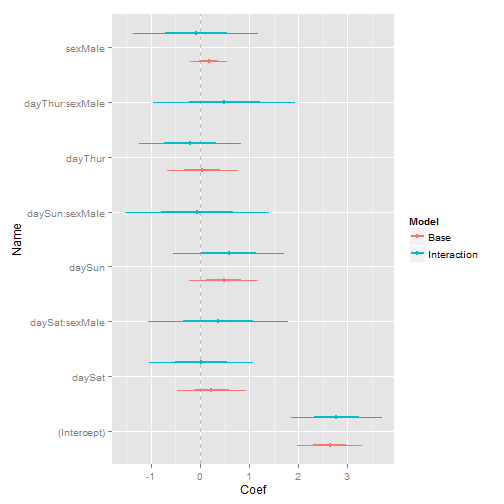Compare that to the multiplot function in coefplot that was built using geom_dodge and coord_flip.

``````multiplot(mod1, mod2, shorten = F, names = c("Base", "Interaction"))
``````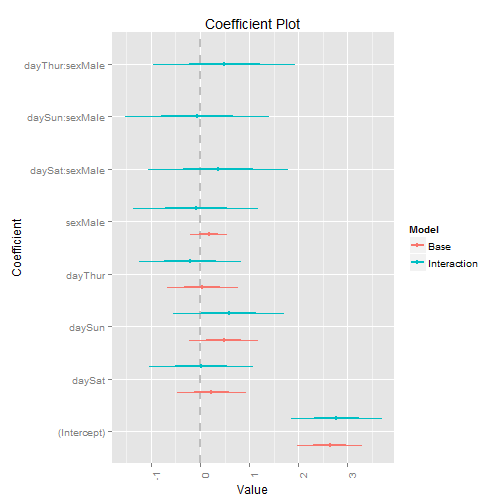With the exception of the ordering and plot labels, these charts are the same. The main benefit here is that avoiding coord_flip still allows the plot to be faceted, which was not possible with coord_flip.

Hopefully Hadley will be able to take these functions and incorporate them into ggplot2.Jared Lander is the Chief Data Scientist of Lander Analytics a New York data science firm, Adjunct Professor at Columbia University, Organizer of the New York Open Statistical Programming meetup and the New York and Washington DC R Conferences and author of R for Everyone.A great way to visualize the results of a regression is to use a Coefficient Plot like the one to the right.  I’ve seen people on Twitter asking how to build this and there has been an option available using Andy Gelman’s coefplot() in the arm package.  Not knowing this I built my own (as seen in this post about taste testing tomatoes) and they both suffered the same problems:.  Long coefficient names often got cut off by the left margin of the graph and the name of the variable was appended to all the levels of a factor.  One big difference between his and mine is that his does not include the Intercept by default.  Mine includes the intercept with the option of excluding it.

I managed to solve the latter problem pretty quickly using some regular expressions.  Now the levels of factors are displayed alone, without being prepended by the factor name.  As for the former, I fixed that yesterday by taking advantage of ggplot by Hadley Wickham which deals with the margins better than I do.

Both of these changes made for a vast improvement over what I had avialable before.  Future improvements will address the sorting of the coefficients displayed and allow users to choose their own display names for the coefficients.

The function is in this file and is called plotCoef() and is very customizable, down to the color and line thickness.  I kept my old version, plotCoefBase(), in the file in case some people are adverse to using ggplot, though no one should be.  I sent the code to Dr. Gelman to hopefully be incorporated into his function which I’m sure gets used by a lot more people than mine will.  Examples of my old version and of Dr. Gelman’s are after the break.

As always, any comments or questions are welcomed.  Go to the Contact page or send an email to contact -at- jaredlander -dot- com or find me on Twitter @jaredlander. Continue readingJared Lander is the Chief Data Scientist of Lander Analytics a New York data science firm, Adjunct Professor at Columbia University, Organizer of the New York Open Statistical Programming meetup and the New York and Washington DC R Conferences and author of R for Everyone.